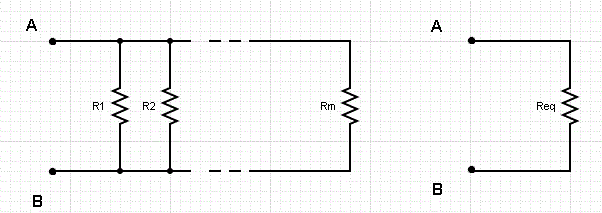# Parallel Resistors Calculator

A parallel resistors calculator that calculates the equivalent resistance is presented. Consider the group of parallel resistors R1, R2, ... Rm in the diagram below. These resistors have an equivalent resistance Req that has the same effects on the other parts of the circuit and is given by the resistors in parallel formula:

1 / Req = 1 / R1 + 1 / R2 + .... + 1 / RmFor example, for 2 resistors R1 and R2 in parallel, Req is given by

1 / Req = 1 / R1 + 1 / R2

When the above is solved for Req, we obtain

Req = (R1 R2) / (R1 + R2)

For 3 resistors R1, R2 and R3 in parallel, Req is given by

1 / Req = 1 / R1 + 1 / R2 + 1 / R3

Solve for Req, to obtain

Req = R1 R2 R3 / (R1 R2 + R1 R3 + R2 R1)

As it can be seen, the calculations beomes complicated as soon as we start increasing the number of parallel resistors in the circuit.

## Use the Parallel Resistors Calculator

Enter the resistances of the resistors R1, R2, ... Rn as positive real numbers separated with commas and then press "Calculate Equivalent Resistance". You may enter any number of resistances separated by commas.

 Resistances of R1, R2, ... Rn: = 4,6,10.5 Ω Decimal Places = 4 Equivalent Resistance: = Ω